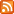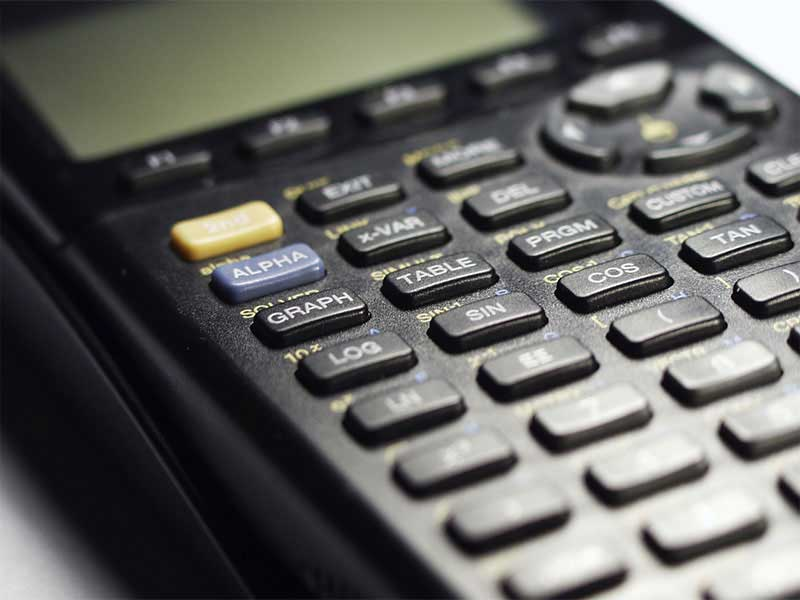ArticlesRSS10 Reasons We Couldn't Live Without Statistics

03.19.22

The discipline of mathematics known as statistics is concerned with the gathering, organization, analysis, and interpretation of sets of numerical data. In the contemporary era of machine learning and big data...

Category: Math Tips For Students and TeachersHappy Pi Approximation Day

07.22.21

July 22nd is "Pi Approximation Day", when all fans of circles, disks and round things in general can celebrate the ratio of the diameter of a circle to its circumference. Sort of. More or less.

Category: Math Tips For Students and TeachersLearning with Graphing Calculators

11.19.16

With the graphing calculator, you are able to have the calculator create graphs which are typically used to solve mathematical equations. Each type of graph which this calculator can solve are typically solved using a graphing calculator, although there are manual ways for them..

Category: Math Tips For Students and TeachersThe Evolution of Calculators

08.27.16

Today, calculators are so ubiquitous that's it's hard to imagine there was a time when their use was largely confined to engineers and academics. Calculators were once strictly stand-alone devices that were quite large by modern standards and limited to a few basic functions.

Category: Math Tips For Students and Teachers